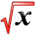# Integration ApplicationsThis article is part of the MathHelp Tutoring Wiki

## Length

QUESTION Find the length of graph of function f(x) = 0.5(ex + e-x)

ANSWER This looks scary, but it's actually just algebra. The formula for arc length is L = int_ab sqrt[1 + (f'(x))2] dx. First we find f'(x) = 0.5(ex - e-x). (f'(x))2 = 0.25(e2x - 2 + e-2x) (this is basically (a-b)2 = a2 - 2ab + b2). By adding 1, the expression becomes 1 + (f'(x))2 = 0.25(e2x + 2 + e-2x). Notice that the only change is the sign for the middle term "2", which gives us something that resembles the original expression except for the sign. As we know that (a+b)^ = a2 + 2ab + b2, taking the square root of 1+(f'(x))2 is 0.5(ex + e-x-x). integrating this we get 0.5(ex - e-x) from a to b (not given in the question).

QUESTION The function int_0x (cost)0.5 dt cannot be expressed with antiderivatives. However we can find the length from x = 0 to x = pi/2.

ANSWER Note that with the arc length formula L = int_a^b sqrt[1 + (f'(x))2] dx. The function f(x) is the whole term int_0^x (cost)0.5 dt (and not (cos t)0.5). We can find f'(x) with fundamental theorem of calculus. f'(x) = (cos x)0.5, so L = int_0^pi/2 sqrt[1 + cos(x)] dx. We can use the double angle formula 1 + cos(x) = 2(cos(x/2))2 (note: this formula is the same as cos2x = 2cos2(x) - 1) to simply the expression and get L = int_0^pi/2 sqrt(2) cos(x/2) dx = 2sqrt(2) sin(x/2) |_0^pi/2 = 2

## Centroid

QUESTION Find the centroid of the semi-ellipse (x/a)2 + (y/b)2 = 1, for x >= 0.

ANSWER The centroid formula is int (x p(x))dx/int p(x) dx, where p(x) is the "height" of the function/shape. For the question here, we have to rearrange our equation to isolate y, ie, y = b sqrt(1 - (x/a)2). This is our p(x). For the integration, we have to do the top and the bottom part separately.

For the top part, we have b* int_0^a x sqrt(1 - (x/a)2) dx. This can be done by standard substitution u = 1 - (x/a)2, which gives du = -(2x/a2) dx. Substituting we get the integral to become -$a^{2}b$/2 int_0^a sqrt(u) du = -a2b/2 (2/3) u3/2 = -a2b/3 (1 - (x/a)2)|_0^a = a2b/3.

For the bottom part, we have to use trig substitution for the integral b*int_0^a sqrt(1 - (x/a)2) dx. If we rearrange sqrt(1 - (x/a)2) to 1/a sqrt(a2 - x2), we can see that this is (clearly) of "a2- x2" form, so we let x = a sin(t). Differentiating to get dx = a cos(t), sub in to see that integral becomes b*int sqrt(cos2 t) *a cos(t) dt = ab*int cos2(t) dt. Using the double angle formula we have ab*int (1 + cos2t)/2 dt = ab/4*[2t + sin(2t)]_0^{pi/2}. Note the limit of integration changes as we made the substitution x = a sin(t). Solving yields, abpi/4.

Divide the top by the bottom integral to get answer x = 4a/3pi.

## Area

QUESTION Let R be the region enclosed by the curves x=1+(y-2)2 and x=2, Sketch R and find its area

  First, we want to find the boundary of the area.
x=1+(y-2)2=2
so (y-2)2=1
y=3 or y=1
Region= integrate from 1 to 3 [2-[1+(y-2)2]]dy


= integrate from 1 to 3(4y-y2-3)dy = [2y2-1/3*y3-3y] from 1 to 3 = 0-(-4/3) =4/3

  In conclusion, R=4/3


## Volume

QUESTION Second, find the volume of the solid obtained by rotating R about the line y=0

 V= integrate from 1 to 3( 2*pi*y[2-(1+(y-2)2)])dy


=2*pi*integrate from 1 to 3 (y*(4y-y2-3)dy = 2*pi* integrate from 1 to 3 (4y2-y3-3y)dy =2*pi*[4/3*y3-1/4*y4-3/2*y2]dy = 2*pi*[4/3*27-1/4*48-3/2*9)-(4/3-1/4-3/2)] = 2*pi*[9/4-(-5/12)] = 2*pi*32/12 =16/3*pi

QUESTION Write the integral for the volume of the solid obtained by rotating R about the y-axis

ANSWER V=inte/ 1 to 3 [pi*(22-[1+(y-2)2]2)]dy

## Work, Spring

QUESTION If 6J of work is needed to stretch a spring from 10cm to 12cm and another 10J of work is needed to stretch it from 12cm to 14cm, what is the natural length of the spring?

Let A be the natural length of the spring.

6=W1=∫ from 10-A to 12-A, (kx)*dx

=1/2*k*(x2) from 10-A to 12-A

=1/2*k*[(12-A)2-(10-A)2]

=1/2*k*(44-4A)

10=W2=integrate from 12-A to 14-A (kx)dx

=1/2*k*[x^2] from 12-A to 14-A

=1/2*k*[(14-A)2-(12-A)2]]=1/2K(52-4A)

So W1/W2=6/10=(44-4A)/(52-4A)

thus 3*(52-4A)=5*(44-4A)

156-12A=220-20A

8A=64

A=8cm

So the natural length of the spring is 8cm.

## Present Value of an Income Stream

Present Value of a continuous income stream Formula :

present value = ∫T2T1 K(t)$e^{rt}$dt
K(t) = dollars per year = annual rate of the income at time t
r = annual interest rate of the money being invested
T1 to T2 = in years, it is the time period of the income stream

Question:The rate of revenue that is produced by a robot at time t is 5000 - 100t dollars per year. What is the present value of this continuous stream of income after 4 years with 16% interest rate.

Solution:

K(t) = 5000 - 100t
T = 4
r = 0.16
present value = ∫40$(5000-100t)e^{-0.16t}$dt
Solve by integration by parts.
(5000 - 100t)$/frac{1}{-0.16}e^{-0.16t}$|40 - ∫40 (-100)${\frac {1}{-0.16}}e^{-0.16t}$dt
= approximately = 16090 - ${\frac {100}{0.16}}$x ${\frac {100}{-0.16}}e^{-0.16t}$|40
= 16090 - 1847
= \$14243